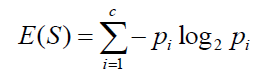top of pageSearch

# Help Needed for a Mom who is homeschooling her kid! Decision Tree-Information Gain-GINI Index

Decision Tree:

A decision tree is a widely used machine learning modeling technique for regression and classification problems in which the data is continuously split according to a certain parameter. The Decision tree has three main components: Root node, Parent nodes and Leaf nodes.

There are different algorithms to build a decision tree such as Information Gain, GINI Index.

Let's get back to the title of this blog where I need your help! Here is my data on which I'm going to apply both Information Gain and GINI Index.In the above chart I have tried to put some of the factors that are affecting the homeschooling of my kid.

‘Homeschool status’ is how productive that day was.

‘Sleep time of kid(More than9 hours)’ is if she slept more than 9 hours or not

‘My schedule’ is my daily work schedule

‘Meal Prep’ is have I done meal prep the previous night

Information Gain:

The information gain is based on the decrease in entropy after a dataset is split on an attribute. Constructing a decision tree is all about finding the attribute that returns the highest information gain (i.e., the most homogeneous branches). The result is the Information Gain, or decrease in entropy.

Entropy:Information Gain : Entropy(parent) - [weighted avg] * entropy(Children)

Entropy of Parent node:

SSFF(Homeschool status) => Parent node

P(Success) = 2/4 = 0.5

=-[0.5 log2(0.5) +0.5 log2(0.5)]

=-[-0.5+(-0.5)]

=1

Entropy of child nodes:

(Sleep time of the Kid)S - Success(Homeschool Status)

F - Failure(Homeschool Status)

Entropy of SF is 1(both the child node)

Formula of Entropy(children) with weighted Avg:

[weighted avg]Entropy(children) = [(no.of examples in left child node)/(Total no.of.examples in parent node) * (entropy of left node)] + [(no.of examples in Right child node)/(Total no.of.examples in parent node) * (entropy of right node)]

Weighted avg(children) = 2/4 * 1 + 2/4 *1

= 1

Information Gain = Entropy(parent) - [weighted avg] * entropy(Children)

= 1-1

= 0

(My Schedule):P(success) = ⅔ =0.667

P(Failure) = ⅓ =0.334

Entropy(Left child node) = -[0.667 log2(0.667) + 0.334 log2(0.334)]

= -[-0.38 + (-0.52)]

= 0.9

Entropy(right child node) = 0

Formula of Entropy(children) with weighted Avg:

[weighted avg]Entropy(children) = [(no.of examples in left child node)/(Total no.of.examples in parent node) * (entropy of left node)] + [(no.of examples in Right child node)/(Total no.of.examples in parent node) * (entropy of right node)]

Weighted avg(children) = ¾ * 0.9 + ¼ *0

= 0.675

Information Gain = Entropy(parent) - [weighted avg] * entropy(Children)

= 1- 0.675

= 0.325

(Meal Prep):P(success) = ⅔ =0.667

P(Failure) = ⅓ =0.334

Entropy(Left child node) = -[0.667 log2(0.667) + 0.334 log2(0.334)]

= -[-0.38 + (-0.52)]

= 0.9

Entropy(right child node) = 0

Formula of Entropy(children) with weighted Avg:

[weighted avg]Entropy(children) = [(no.of examples in left child node)/(Total no.of.examples in parent node) * (entropy of left node)] + [(no.of examples in Right child node)/(Total no.of.examples in parent node) * (entropy of right node)]

Weighted avg(children) = ¾ * 0.9 + ¼ *0

= 0.675

Information Gain = Entropy(parent) - [weighted avg] * entropy(Children)

= 1- 0.675

= 0.325

Conclusion(Information Gain):

Information Gain(Sleep time of the Kid) = 0

Information Gain(My Schedule) = 0.325

Information Gain(Meal Prep) = 0.325

GINI Index:

Gini Index, also known as Gini impurity, calculates the amount of probability of a specific feature that is classified incorrectly when selected randomly. If all the elements are linked with a single class then it can be called pure.Where Pi denotes the probability of an element being classified for a distinct class.

Gini Index for ‘Sleep time of Kid(hours):

P(yes)= 2/4

P(no)= 2/4

If(Sleep time of kid(More than 9 hours) = Yes & Homeschool status = Success): 1/2

If(Sleep time of kid(More than 9 hours) = Yes & Homeschool status = Failure): ½

Gini Index(yes)= 1- [(½)^2+(1/2)^2]

=1-[0.25+0.25]

= 0.5

If(Sleep time of kid(More than 9 hours) = No & Homeschool status = Success): 1/2

If(Sleep time of kid(More than 9 hours) = No & Homeschool status = Failure): ½

Gini Index(No) = 1- [(½)^2+(1/2)^2]

=1-[0.25-0.25]

= 0.5

Gini Index(Sleep Time of Kid) = 2/4 * 0.5 + 2/4 * 0.5

= 0.25+0.25

= 0.5

Gini Index for ‘My Schedule’:

P(Not busy)= ¾

P(Busy) =¼

If(My Schedule = Not Busy & Homeschool Status = Success): 2/3

If(My Schedule = Not Busy & Homeschool Status = Failure): 1/3

Gini Index(Not Busy) = 1-[(⅔)^2+(⅓)^2]

= 1 -(0.44+0.11)

= 0.45

If(My Schedule = Busy & Homeschool Status = Success): 0

If(My Schedule = Busy & Homeschool Status = Failure): 1

Gini Index(Busy) = 1-[(0)^2+(1)^2]

= 0

Gini Index(My Schedule) = ¾*0.45 + ¼*0

= 0.3375

Gini Index for ‘Meal Prep’:

P(Yes)= ¾

P(No) =¼

If(Meal Prep = Yes & Homeschool Status = Success): 2/3

If(Meal Prep = Yes & Homeschool Status = Failure): 1/3

Gini Index(Yes) = 1-[(⅔)^2+(⅓)^2]

= 1 -(0.44+0.11)

=0.45

If(Meal Prep = No & Homeschool Status = Success): 0

If(Meal Prep = No & Homeschool Status = Failure): 1

Gini Index(No) = 1-[(0)^2+(1)^2]

= 0

Gini Index(Meal Prep) = ¾*0.45 + ¼*0

= 0.3375

Conclusion(GINI Index):

Gini Index(Sleep Time of Kid) : 0.5

Gini Index(My Schedule) : 0.3375

Gini Index(Meal Prep) : 0.3375

As I said in the title, here comes the problem:

I get the same value for both ‘My Schedule’ and ‘Meal Prep’ in Information Gain and GINI Index. For which should I give more priority to have a successful homeschool for my kid? Help me out!

Happy Learning:)